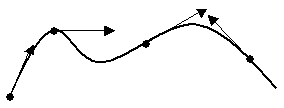# Specifying a Direction

Some commands require a specified a direction or vector for the command. A direction is basically a xyz vector in the model. The following options determine a direction specification:

## Vector (XYZ values)

[Vector] <xval yval zval>

The most basic way to specify a direction is to just give the vector x-y-z components of the direction. The given vector need not be a unit vector. The following three commands simply draw a direction in the x-direction (1, 0, 0) as the Vector keyword is optional and unit vectors are not required:

draw direction vector 1 0 0
draw direction 1 0 0
draw direction 10 0 0

## Last Direction Used

Last

The last option recalls the last direction used in a command. For example, if the following command is entered after the above vector commands a direction location would be drawn in the x-direction (1, 0, 0).

draw direction last

Last directions do not carry over from CUBIT session to CUBIT session. The last direction defaults to (1, 0, 0) if no direction has been used during the session.

## Positive or Negative X,Y,Z Direction Vectors

X|Y|Z|Nx|Ny|Nz

The x|y|z|nx|ny|nz options assign the x direction, y direction, z direction, negative x direction, negative y direction and negative z direction respectively.

## On Curve Tangent

[On] | [Tangent] [At] Curve <id> {location on curve options}

The curve option simply finds a tangent vector on a curve. Note that the on, tangent and at keywords are optional, as well as the location on the curve. If no location is specified, the tangent at the start vertex of the curve is found. See the section above, Specifying a Location on a Curve, for details on how to specify where along the curve the tangent vector is found.

draw direction curve 1
draw direction on curve 1
draw direction tangent at curve 1
draw direction tangent at curve 1 distance 3
draw direction tangent at curve 1 fraction .5
draw direction tangent at curve 1 distance 2 reverseFigure 1 - Tangents to a Curve

## On Surface Normal

[On] | [Normal] [At] Surface <id> [Location {options}]

The surface option simply finds a normal vector on a surface. Note that the "on", "normal" and "at" keywords are optional, as well as the location on the surface. If no location is specified, the normal vector at the center of the surface is found. If a location is specified, the location is projected to the surface, then the normal vector is found.

draw direction on surface 1
draw direction on surface 1 location 1 2 0

## From Location

[From] {Location {options} | Node|Vertex <id>} [Project] {Location {options} | [Entity]
{Node|Vertex|Curve|Surface} <id>}

The from location option finds a direction that is from one location to another or from a location to an entity. If the second specification is an entity, the first location is projected to the entity to find the direction.

draw direction from vertex 1 vertex 2
draw direction from location on curve 1 fraction .5 surface 3

Note that when using an entity for the second specification, the Project and Entity keywords are generally optional. However, it is sometimes necessary to remove ambiguity from the previous location specification. For example, the following will not parse correctly:

draw direction location on curve 1 distance 2 surface 3

In this case, the location on the curve is parsed as a distance 2.0 from surface 3. Instead, the desired behavior is to find the location on curve 1 as a distance of 2.0 along the curve from the start of the curve, and project it to surface 3 to find the direction. The following commands (all equivalent) achieve this behavior:

draw direction location on curve 1 distance 2 project surface 3
draw direction location on curve 1 distance 2 entity surface 3
draw direction location on curve 1 distance 2 project entity surface 3

## Rotate

[Rotate {options}]

The rotate option allows you to rotate the direction about another vector. You can string together as many rotations as necessary. For example:

draw direction 1 0 0 rotate about z 135 rotate about curve 1 angle 50

Options that can be used with rotate are as follows:

{Ax|X|Ay|Y|Az|Z [Angle] <angle>} | { {[About] | Towards} Direction {options} Angle <val> } [Rotate (options)] [Origin (location)]

Ax, Ay, Az (or X,Y,Z) angles can be entered in any order. The optional specification of another rotate keyword in the options indicated that multiple nested rotations are permitted.

## Cross

[Cross [With] Direction {options}]

The cross option allows you to find the vector cross product of the direction with another direction.

## Reverse

[Reverse]

This keyword simply reverses the direction specification.

## Previewing a Direction

Sometimes it is helpful to preview a direction before using it in a command. A direction may be previewed using the Draw command. The direction options are described above. See Specifying a Location for a list of location options.

Draw Direction {direction_options} [Location (location_options)]